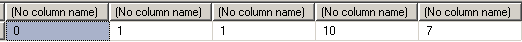﻿ Fuzzy String Matching using Levenshtein Distance Algorithm in SQL ServerSQL Server and T-SQL Development Tutorials Development resources, articles, tutorials, code samples, tools and downloads for ASP.Net, SQL Server, Reporting Services, T-SQL, Windows, AWS, SAP HANA and ABAP

# Fuzzy String Matching using Levenshtein Distance Algorithm in SQL Server

The Levenshtein distance algoritm is a popular method of fuzzy string matching.
Levenshtein distance algorithm has implemantations in SQL Server also.
Levenshtein distance sql functions can be used to compare strings in SQL Server by t-sql developers.

The term Levenshtein distance between two strings means the number of character replacements or chararacter insert or character deletion required to transform one string to other.

Levenshtein distance is also known as Edit Distance.

If two strings are equal the Levenstein distance is 0, zero.
A zero value for Levenshtein distance between two string variables in SQL Server means, these two string variables are identical.

The higher the value of Levenstein distance between two varchar or nvarchar string variables means the strings are more different than each other. As the Levenstein distance algoritm counts each character edition to transform one string to other, if strings are completely different then the Levenstein distance function will result high values.

The return of a SQL Levenstein distance function is an integer.

The name Levenshtein is for the memory of Vladimir Levenshtein who is the developer of this idea.

One of the most used SQL Levenshtein distance among sql programmers is as follows:
Please note that the code is taken from a forum post at SQLTeam.

Please note that this sql function is developed by Joseph Gama.

Here is the outputs of sample Levenshtein distance sql function for SQL Server developers.SQL Server